## Abstract

A theoretical study on free vibration behavior of pre-stressed functionally graded material (FGM) beam is carried out. Power law variation of volume fraction along the thickness direction is considered. Geometric non-linearity is incorporated through von Kármán non-linear strain–displacement relationship. The governing equation for the static problem is obtained using minimum potential energy principle. The dynamic problem for the pre-stressed beam is formulated as an eigenvalue problem using Hamiltons principle. Three classical boundary conditions with immovable ends are considered for the present work, namely clamped–clamped, simply supported–simply supported and clamped–simply supported. Four different FGM beams, namely Stainless Steel–Silicon Nitride, Stainless Steel–Zirconia, Stainless Steel–Alumina and Titanium alloy–Zirconia, are considered for generation of results. Numerical results for non-dimensional frequency parameters of undeformed beam are presented. The results are presented in non-dimensional pressure-displacement plane for the static problem and in non-dimensional frequency-displacement plane for the dynamic problem. Comparative frequency-displacement plots are presented for different FGMs and also for different volume fraction indices.

## Keywords

Functionally graded material ; Large deflection ; Timoshenko beam ; Pre-stressed beam ; Loaded natural frequency

## 1. Introduction

Functionally graded materials (FGMs) are inhomogeneous composites that have smooth and continuous variation of material properties in space. In most of the existing and potential future applications, FGM is considered mainly as a mixture of ceramic and metal in varying proportion. With the strength and toughness of metals, and the thermal and wear resistance of ceramics, FGM components possess good qualities of both the metals and ceramics. This makes it suitable for the FGM structures or components to be used in high temperature environment. FGM components are found in various applications, such as in aerospace, nuclear, automotive, civil, biomechanical, optical, electronic, mechanical, chemical and shipbuilding industries  . FGM components have applications in astronautic structures, such as rocket launch-pad, space vehicles  , etc., because rocket launch-pad is subjected to tremendous thermal and mechanical loading, whereas, space vehicles are subjected to extreme thermal conditions. FGMs having excellent thermal and mechanical properties are suitable for such various astronautic structures. It is to be mentioned that the present work deals with FGM beams, which are often found in various structures in the fields of aerospace, mechanical, automotive, civil engineering, etc.

FGM beams are mainly designed for applications under thermal environment. But its behavior under mechanical loadings at ambient condition is also important in order to ascertain its performance when thermal loadings are absent. Knowledge of free vibration behavior of pre-stressed FGM beams under mechanical loading is important from design point of view. It is known that the amplitude of forced vibration becomes excessively large when the excitation frequency falls in the vicinity of the natural frequency of vibration of a loaded beam. To avoid such undesirable vibration levels, the natural frequency of vibration of the loaded beam must be known to the designer. Hence the present work is meant to investigate such dynamic behavior of FGM beams. The literature review of some related works by other notable researchers are given in the next few paragraphs.

Ke et al.  investigated the nonlinear vibration behavior of FGM beams based on Euler–Bernoulli beam theory and von Kármán geometric nonlinearity. Fallah and Aghdam   and  presented large amplitude free vibration analysis of FGM Euler–Bernoulli beams resting on nonlinear elastic foundation subjected to both mechanical and thermal loadings. Fu et al.  carried out nonlinear free vibration analysis of piezoelectric FGM beams under thermal environment employing Euler–Bernoulli beam theory. Lai et al.  obtained the accurate analytical solutions for large amplitude vibration of thin FGM beams using Euler–Bernoulli beam theory. Based on Euler–Bernoulli beam theory, Yaghoobi and Torabi  studied the nonlinear vibration behavior of geometrically imperfect FGM beams resting on nonlinear elastic foundation subjected to axial force. Hemmatnezhad et al.  studied the large-amplitude oscillations of FGM Timoshenko beams using finite element formulation. Rahimi et al.  performed free vibration analysis of FGM Timoshenko beams in the vicinity of a buckled equilibrium configuration.

Kapuria et al.  presented a theoretical finite element model for vibration analysis of layered FGM beams with experimental validation. Aydogdu and Taskin  studied free vibration behavior of simply supported FGM beams using different beam theories. Free vibration characteristics of simply supported FGM beams were investigated by Şimşek and Kocatürk  using Lagranges equations under the assumptions of the Euler–Bernoulli beam theory. Thermo-mechanical vibration analysis of FGM beams resting on variable elastic foundation was carried out by Pradhan and Murmu  . Free vibration analysis of FGM beams based on a different first order shear deformation theory was carried out by Sina et al.  . Fundamental frequency analysis of FGM beams was carried out by Şimşek  using different higher-order beam theories. Giunta et al.  addressed free vibration behavior of functionally graded beams via several axiomatic refined theories. Using finite element method, Alshorbagy et al.  presented the free vibration characteristics of FGM beams with material graduation axially or transversally through the thickness based on the power law.

Free vibration characteristics of layered functionally graded beams were studied by Wattanasakulpong et al.  using Ritz method. Thai and Vo  investigated free vibration behavior of FGM beams based on various higher-order beam theories. Free vibration analysis of FGM beams for different boundary conditions was carried out by Pradhan and Chakraverty  using Euler–Bernoulli and Timoshenko beam theories. Free vibration behavior of axially loaded rectangular FGM beams was investigated by Nguyen et al.  based on the first-order shear deformation beam theory. The dynamic stiffness method was used by Su et al.  to investigate the free vibration behavior of FGM beams. Wattanasakulpong and Mao  investigated the dynamic response of Timoshenko FGM beams supported by various classical and non-classical boundary conditions.

Esfahani et al.  studied free vibration behavior of a thermally pre/post buckled FGM beam resting over a nonlinear hardening elastic foundation. Free vibration behavior of a thermo-electrically post-buckled rectangular FGM piezoelectric beams was studied by Komijani et al.  . Thermal buckling analysis of FGM beams with temperature-dependent material properties was carried out by Kiani and Eslami   and  . Esfahani et al.  carried out non-linear thermal stability analysis of temperature-dependent FGM beams resting on non-linear hardening elastic foundation. Thermo-electrical stability analysis of piezoelectric FGM beams had been carried out by Kiani et al.  , Kargani et al.  and Komijani et al.  , whereas thermal stability analysis of piezoelectric FGM beams was carried out by Kiani et al.  .

The present work is based on Timoshenko beam theory, which considers uniform distribution of transverse shear stress across the beam thickness. It is worthwhile to mention some of the research works using higher shear deformation theories (HSDT) developed in the recent years for analysis of plate and beam structures. Tounsi et al.  carried out thermo-elastic bending analysis of functionally graded sandwich plates using a refined trigonometric shear deformation theory (RTSDT). The thermo-mechanical bending behavior of FGM plates resting on Winkler–Pasternak elastic foundations was studied by Bouderba et al.  using RTSDT. Buckling and free vibration behaviors of exponentially graded sandwich plates were investigated by Ait Amar et al.  using simple refined shear deformation theory. Static and dynamic analyses of FGM and sandwich plates had been carried out by Hebali et al.  and Mahi et al.  using new hyperbolic shear deformation theory. Using higher-order shear deformation theories, wave propagation analysis in porous FGM plates, and bending and vibration analysis of FGM plates, were carried out by Ait Yahia et al.  and Belabed et al.  respectively. Recently, Bourada et al.  developed a refined trigonometric higher-order beam theory to investigate static and dynamic behaviors of FGM beams. In that work, the authors have included stretching deformation effect along the thickness direction and eliminated the need of shear correction factor. Bousahla et al.  presented a new trigonometric higher-order theory for the static analysis of FGM plates employing the physical neutral surface concept. Hamidi et al.  presented a sinusoidal plate theory for the thermo-mechanical bending analysis of functionally graded sandwich plates. Bessaim et al.  developed a new higher-order shear and normal deformation theory for investigating the bending and free vibration behavior of sandwich plates with functionally graded isotropic face sheets. Thermo-elastic bending analysis of functionally graded sandwich plates was carried out by Bouchafa et al.  using a refined hyperbolic shear deformation theory. Houari et al.  , using a new higher-order shear and normal deformation theory, simulated the thermo-elastic bending of FGM sandwich plates.

From the literature review presented, it is clear that an exhaustive study on free vibration behavior of transversely loaded beam for different FGM materials and different classical boundary conditions is scarce. Most of the published works are involved with either free vibration behavior of undeformed FGM beam or large amplitude free vibration behavior of FGM beam. Hence, in the present work, free vibration frequencies of FGM beam are computed for different pre-stressed configurations under uniform transverse pressure. Pre-stressed configurations are obtained through a geometrically non-linear static analysis. The linear vibration frequency of the pre-stressed beam, hereafter termed as loaded natural frequency, is then computed through an eigenvalue problem that includes the effect of pre-stressing using the displacement fields of the static problem. The effect of geometric non-linearity is included using von Kármán non-linear strain–displacement relationship. Timoshenko beam theory is used to consider the effects of shear deformation for the static problem and of rotary inertia for the subsequent dynamic problem. Suitable energy-based variational principles are used to derive the governing equations for both parts of the problem. Four different functionally graded materials and three different immovable classical boundary conditions are considered to show the pre-stressed dynamic behavior of beams.

## 2. Mathematical formulation

The present work aims at finding loaded natural frequency of pre-stressed FGM Timoshenko beam. A uniform rectangular beam with length L , height h and width b is considered. A beam with symbolic dimensions is shown in Fig. 1 , where, x , y and z denote the coordinate axes along the length, width and thickness directions respectively. As mentioned earlier, two distinct but interrelated problems are formulated and solved to obtain the desired solution. The purpose of the first one, the static problem, is to obtain the pre-stressed configuration of the beam under the application of uniform transverse pressure. And the second problem, named as the dynamic problem, is utilized to obtain the loaded natural frequency of the deformed beam. It must be mentioned that the static configurations for different loadings are obtained through a geometrically non-linear analysis to address the large deflection effect.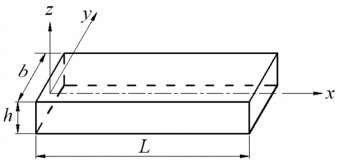Fig. 1. Beam with dimensions and coordinate axes.

### 2.1. Modeling of FGM beam

The present work considers continuous variation of graded material properties across the beam thickness. A continuous variation of volume fraction of ceramic (${\textstyle V_{c}}$ ) and metal (${\textstyle V_{m}}$ ) constituents across the thickness is assumed in accordance with the power-law given by ${\textstyle V_{c}={\left({\frac {z}{h}}+{\frac {1}{2}}\right)}^{k}}$ and ${\textstyle V_{m}=1-V_{c}}$ respectively  . Here, ${\textstyle k{\mbox{ }}\left(0\leq k\leq \infty \right)}$ is the volume fraction index. Hence the effective material property ${\textstyle P_{f}}$ of any FGM layer is determined using the Voigt model, which is given by ${\textstyle P_{f}=P_{c}V_{c}+P_{m}V_{m}}$ , where ${\textstyle P_{c}}$ and ${\textstyle P_{m}}$ are the material properties of the ceramic and metal constituents respectively. So any effective material property at any layer z   is given by ${\textstyle P_{f}{\mbox{ }}\left(z\right)=P_{m}+\left(P_{c}-P_{m}\right){\left({\frac {z}{h}}+{\frac {1}{2}}\right)}^{k}}$ . For the present FGM beam model, the top layer (${\textstyle z=+h/2}$ ) is purely ceramic and the bottom layer (${\textstyle z=-h/2}$ ) is purely metal. It is to be mentioned that the subscripts m and c refer to the metal and ceramic constituents respectively.

Any material property of the individual constituent is temperature-dependent and such temperature dependence is considered using the relationship ${\textstyle P_{c}{\mbox{ }}{\mbox{or}},{\mbox{ }}P_{m}=P_{0}{\mbox{ }}\left[P_{-1}T^{-1}+\right.}$$\left.1+P_{1}T+P_{2}T^{2}+P_{3}T^{3}\right]$ , where ${\textstyle T}$ is the temperature in K and ${\textstyle P_{0}}$ , ${\textstyle P_{-1}}$ , ${\textstyle P_{1}}$ , ${\textstyle P_{2}}$ and ${\textstyle P_{3}}$ are the coefficients of temperature. The relevant effective material properties for the present problem are elastic modulus ${\textstyle E_{f}}$ , shear modulus ${\textstyle G_{f}}$ , Poissons ratio ${\textstyle {\upsilon }_{f}}$ and density ${\textstyle {\rho }_{f}}$ . The temperature coefficients  of the material properties for the ceramic or metal constituents considered are given in Table 1 . For the present problem, the beam is assumed to be at ambient temperature ${\textstyle T_{0}=300{\mbox{ }}K}$ , which is also considered to be the temperature at which thermal stress is zero. Hence all the required material properties are calculated at ${\textstyle T_{0}}$ .

Table 1. Temperature coefficients of FGM constituents.
Constituent material Property
 $P_{0}$

 $P_{-1}$

 $P_{1}$

 $P_{2}$

 $P_{3}$

SUS304 ${\textstyle E}$ (Pa) 201.04 × 109 0 3.079 × 10−4 −6.534 × 10−7 0
 $\upsilon$

0.3262 0 −2.002 × 10−4 3.797 × 10−7 0
${\textstyle \rho }$ (kg m−3 ) 8166 0 0 0 0
Ti-6Al-4V ${\textstyle E}$ (Pa) 122.56 × 109 0 −4.586 × 10−4 0 0
 $\upsilon$

0.2884 0 1.121 × 10−4 0 0
${\textstyle \rho }$ (kg m−3 ) 4429 0 0 0 0
Si3 N4 ${\textstyle E}$ (Pa) 348.43 × 109 0 −3.070 × 10−4 2.160 × 10−7 −8.946 × 10−11
 $\upsilon$

0.2400 0 0 0 0
${\textstyle \rho }$ (kg m−3 ) 2370 0 0 0 0
ZrO2 ${\textstyle E}$ (Pa) 244.27 × 109 0 −1.371 × 10−3 1.214 × 10−6 −3.681 × 10−10
 $\upsilon$

0.2882 0 1.133 × 10−4 0 0
${\textstyle \rho }$ (kg m−3 ) 3000 0 0 0 0
Al2 O3 ${\textstyle E}$ (Pa) 349.55 × 109 0 −3.853 × 10−4 4.027 × 10-7 −1.673 × 10−10
 $\upsilon$

0.2600 0 0 0 0
${\textstyle \rho }$ (kg m−3 ) 3750 0 0 0 0

### 2.2. Static problem

The governing equation of the static problem is obtained using the minimum potential energy principle  given by,

 $\delta \left(U+V\right)=0$
( 1)

where ${\textstyle U}$ is the strain energy developed due to external loadings, ${\textstyle V}$ is the potential energy of the external loadings, and ${\textstyle \delta }$ is the variational operator. The strain energy ${\textstyle U}$ consists of two parts, i.e., ${\textstyle U=U_{ax}+U_{sh}}$ , where ${\textstyle U_{ax}}$ and ${\textstyle U_{sh}}$ are the strain energies due to axial strain and shear strain respectively. Using the proportionality of stress and strain (i.e., linear elastic material), and using suitable strain–displacement relationships, the strain energies can be expressed in terms of the displacement fields. The three displacement fields considered for the present problem are the following: ${\textstyle u}$ , the in-plane displacement field, ${\textstyle w}$ , the transverse displacement field, and ${\textstyle \psi }$ , the rotational field of beam cross section due to bending. Here ${\textstyle u}$ , ${\textstyle w}$ and ${\textstyle \psi }$ are defined at the mid-plane of the beam and are functions of the axial coordinate x .

The expressions of axial strain and shear strain are given by,

 ${\epsilon }_{ax}={\frac {1}{2}}{\left({\frac {dw}{dx}}\right)}^{2}+$${\frac {du}{dx}}-z{\frac {d\psi }{dx}}$
( 2)

and

 ${\epsilon }_{sh}={\frac {1}{2}}\left({\frac {dw}{dx}}-\psi \right)$
( 3)

It is to be mentioned that the first term of Eq. (2) is von Kármán type non-linear strain–displacement relationship. Hence the strain energies ${\textstyle U_{ax}}$ and ${\textstyle U_{sh}}$ are given by,

 $U_{ax}={\frac {1}{2}}\int _{0}^{L}\lbrace {\frac {A_{ax}}{4}}{\left({\frac {dw}{dx}}\right)}^{4}+A_{ax}{\mbox{ }}{\left({\frac {du}{dx}}\right)}^{2}+C_{ax}{\mbox{ }}{\left({\frac {d\psi }{dx}}\right)}^{2}$ ${\mbox{ }}+{\mbox{ }}A_{ax}{\mbox{ }}{\left({\frac {dw}{dx}}\right)}^{2}{\frac {du}{dx}}-$$B_{ax}{\mbox{ }}{\left({\frac {dw}{dx}}\right)}^{2}{\frac {d\psi }{dx}}-$$2B_{ax}{\frac {du}{dx}}{\frac {d\psi }{dx}}\rbrace dx$
( 4)

and

 $U_{sh}={\frac {k_{sh}}{2}}\int _{0}^{L}\left\{A_{sh}{\mbox{ }}{\left({\frac {dw}{dx}}\right)}^{2}-2A_{sh}{\frac {dw}{dx}}\psi +A_{sh}{\psi }^{2}\right\}dx$
( 5)

The stiffness coefficients used in Eqs. (4) and (5) are given below:

${\textstyle \left(A_{ax}{\mbox{​}},{\mbox{ }}B_{ax}{\mbox{​}},{\mbox{ }}C_{ax}\right)=}$$b\int _{-{\frac {h}{2}}}^{+{\frac {h}{2}}}E_{f}{\mbox{ }}\left(1,{\mbox{ }}z,{\mbox{ }}z^{2}\right)dz$ and ${\textstyle A_{sh}=b\int _{-{\frac {h}{2}}}^{+{\frac {h}{2}}}G_{f}dz}$ . In Eq. (5) , ${\textstyle k_{sh}}$ is the shear correction factor, which is taken to be 5/6 for rectangular cross section. It is to be mentioned that the shear modulus ${\textstyle G_{f}}$ is given by, ${\textstyle G_{f}={\frac {E_{f}}{2\left(1+\upsilon f\right)}}}$ .

The potential energy of the applied uniform transverse pressure ${\textstyle p}$ is given by,

 $V=-\int _{0}^{L}pwdx$
( 6)

where ${\textstyle p}$ is defined as the force per unit length of the beam.

Following Ritz method, the displacement fields are approximated as finite linear combinations of admissible functions and unknown coefficients given as,

 $w=\sum _{i=1}^{nw}d_{i}{\phi }_{i}{\mbox{​}},{\mbox{ }}u=$$\sum _{i=1}^{nu}d_{nw+i}{\alpha }_{i}{\mbox{​}},{\mbox{ }}\psi =$$\sum _{i=1}^{nsi}d_{nw+nu+i}{\beta }_{i}$
( 7)

Here, ${\textstyle {\phi }_{i}}$ , ${\textstyle {\alpha }_{i}}$ and ${\textstyle {\beta }_{i}}$ are set of orthogonal admissible functions for the displacement fields${\textstyle w}$ , ${\textstyle u}$ and ${\textstyle \psi }$ respectively; and ${\textstyle nw}$ , ${\textstyle nu}$ and ${\textstyle nsi}$ are the number of functions used to approximate ${\textstyle w}$ , ${\textstyle u}$ and ${\textstyle \psi }$ respectively. It is to be noted that ${\textstyle d_{i}}$ is the set of unknown coefficients, which are to be determined from the governing equations. The admissible functions satisfy the boundary conditions of the beam. The lowest order functions for each of the displacement fields are selected suitably and the corresponding higher-order functions are developed numerically following Gram–Schmidt orthogonalization scheme. Three boundary conditions with immovable ends are considered for the present work. And these are clamped–clamped (CC), simply supported–simply supported (SS) and clamped–simply supported (CS). The selected lowest order admissible functions for each of the displacement fields are given in Table 2 for all the three boundary conditions considered.

Table 2. List of lowest order admissible functions for the displacement fields.
Displacement field Boundary Conditions Function
 $w$

CC
 ${w\vert }_{x=0}=0,{\mbox{ }}{w\vert }_{x=L}=0$

 ${\phi }_{1}=\left(x/L\right)\left\{1-\left(x/L\right)\right\}$

SS
CS
 $u$

CC
 ${u\vert }_{x=0}=0,{\mbox{ }}{u\vert }_{x=L}=0$

 ${\alpha }_{1}=\left(x/L\right)\left\{1-\left(x/L\right)\right\}$

SS
CS
 $\psi$

CC
 ${\psi \vert }_{x=0}=0,{\mbox{ }}{\psi \vert }_{x=L}=$$0$

 ${\beta }_{1}=sin\left(\pi x/L\right)$

SS
 ${\psi \vert }_{x=0}\not =0,{\mbox{ }}{\psi \vert }_{x=L}\not =$$0$

 ${\beta }_{1}=cos\left(\pi x/L\right)$

CS
 ${\psi \vert }_{x=0}=0,{\mbox{ }}{\psi \vert }_{x=L}\not =$$0$

 ${\beta }_{1}=sin\left(\pi x/\left(2L\right)\right)$

Using the expression of various potential energies, given by Eqs. (4) , (5) and (6) into Eq. (1) and using the approximate displacement fields, given by Eq. (7) , the governing algebraic equations are obtained in the form given below:

 $\left[K_{\mbox{ij}}\right]\left\{d_{j}\right\}=\left\{f_{i}\right\}$
( 8)

where ${\textstyle \left[K_{\mbox{ij}}\right]}$ and ${\textstyle \left\{f_{i}\right\}}$ are the stiffness matrix and load vector, respectively, each of dimension ${\textstyle nu+nw+nsi}$ . The elements of ${\textstyle \left[K_{\mbox{ij}}\right]}$ and ${\textstyle \left\{f_{i}\right\}}$ are given in the Appendix. It can be seen that the set of governing equations is non-linear in nature as the stiffness matrix is a function of the unknown coefficients. To solve this set of non-linear equations, a multi-dimensional secant method known as Broydens method   and  is used. The solution of Eq. (8) gives the statically deflected configuration of a pre-stressed beam. The next stage of the problem is now to determine the loaded natural frequency of the pre-stressed beam and its mathematical formulation is discussed in the next section.

### 2.3. Dynamic problem

The governing equation of the dynamic problem is derived using Hamiltons principle  given by,

 $\delta \left(\int _{t_{1}}^{t_{2}}\left(T_{k}-U-V\right)dt\right)=$$0$
( 9)

where ${\textstyle T_{k}}$ is the kinetic energy of the vibrating beam and ${\textstyle t}$ is the time. The present work is a free vibration problem of a pre-stressed beam, in which the pre-stressed configuration is already obtained in the previous step of static problem. Hence the potential energy ${\textstyle V}$ of the external loadings is zero in this case. Taking ${\textstyle {\rho }_{f}}$ as the effective density of any FGM layer, the expression of ${\textstyle T_{k}}$ is as follows:

 $T_{k}={\frac {1}{2}}\int _{0}^{L}\left\{D{\left({\frac {dw}{dt}}\right)}^{2}+D{\left({\frac {du}{dt}}\right)}^{2}+F{\left({\frac {d\psi }{dt}}\right)}^{2}\right\}dx$
( 10)

where the inertia coefficients ${\textstyle D}$ and ${\textstyle F}$ are given by ${\textstyle \left(D,{\mbox{ }}F\right)=b\int _{-{\frac {h}{2}}}^{+{\frac {h}{2}}}{\rho }_{f}{\mbox{ }}\left(1,{\mbox{ }}z^{2}\right)dz}$ . The expression of ${\textstyle U}$ remains the same as given for the static problem.

The approximate dynamic displacement fields, which are assumed to be separable in space and time, are given by,

 $w\left(x,{\mbox{ }}t\right)=\sum _{i=1}^{nw}d_{i}{\phi }_{i}{\mbox{ }}\left(x\right)e^{i\omega t}{\mbox{​}},$ $u\left(x,{\mbox{ }}t\right)=\sum _{i=1}^{nu}d_{nw+i}{\alpha }_{i}{\mbox{ }}\left(x\right)e^{i\omega t}{\mbox{​}},$ $\psi \left(x,{\mbox{ }}t\right)=\sum _{i=1}^{nsi}d_{nw+nu+i}{\beta }_{i}{\mbox{ }}\left(x\right)e^{i\omega t}{\mbox{​}},$
( 11)

where ${\textstyle i={\sqrt {-1}}}$ and ${\textstyle d_{i}}$ are a new set of unknown parameters for the dynamic problem. The complete set of the space part of the dynamic displacements, i.e., ${\textstyle {\phi }_{i}}$ , ${\textstyle {\alpha }_{i}}$ and ${\textstyle {\beta }_{i}}$ , is the same as taken for the static problem. In Eq. (11) , ${\textstyle \omega }$ denotes the natural frequency of vibration of the beam.

Using the expressions of strain energy (Eqs. (4) and (5) ), kinetic energy (Eq. (10) ) and dynamic displacements (Eq. (11) ), the governing equation is obtained as follows:

 $\left[K_{\mbox{ij}}\right]\left\{d_{j}\right\}-{\omega }^{2}{\mbox{ }}\left[M_{\mbox{ij}}\right]\left\{d_{j}\right\}=$$0$
( 12)

where ${\textstyle \left[K_{\mbox{ij}}\right]}$ and ${\textstyle \left[M_{\mbox{ij}}\right]}$ are the stiffness matrix and mass matrix respectively. The elements of ${\textstyle \left[K_{\mbox{ij}}\right]}$ are the same as given in the Appendix and the elements of ${\textstyle \left[M_{\mbox{ij}}\right]}$ are given below:

 ${\left[M_{\mbox{ij}}\right]}_{\begin{array}{c}i=1,nw\\j=1,nw\end{array}}=D\int _{0}^{L}{\phi }_{i}{\phi }_{j}{\mbox{dx}},$ ${\left[M_{\mbox{ij}}\right]}_{\begin{array}{c}i=nw+1,nw+nu\\j=nw+1,nw+nu\end{array}}=D\int _{0}^{L}{\alpha }_{i-nw}{\alpha }_{j-nw}{\mbox{dx}},$ ${\left[M_{\mbox{ij}}\right]}_{\begin{array}{c}i=nw+nu+1,nw+nu+nsi\\j=nw+nu+1,nw+nu+nsi\end{array}}=F\int _{0}^{L}{\beta }_{i-nw-nu}{\beta }_{j-nw-nu}{\mbox{dx}}.$
( 13)

The off-diagonal elements of ${\textstyle \left[M_{\mbox{ij}}\right]}$ are zero. Eq. (12) is an eigenvalue problem, in which the square root of the eigenvalues gives the natural frequency of vibration of various vibration modes and ${\textstyle \left\{d_{j}\right\}}$ is the corresponding eigen vectors used to obtain the vibration mode shapes. It must be noted that the stiffness matrix given in Eq. (12) is non-linear in nature. But as the dynamic problem is to be formulated at the pre-stressed beam configuration, the non-linear terms of the stiffness matrix are updated with the pre-stressed beam displacement fields obtained from the static problem  . Hence the solution of Eq. (12) gives the linear loaded natural frequency of vibration of the pre-stressed beam.

## 3. Results and discussion

The present work is carried out to determine the natural frequency of the first mode of vibration of pre-stressed FGM beams. It is to be mentioned that the frequency determined is of small amplitude vibration of pre-stressed beam. So the dynamic behavior is presented graphically in non-dimensional ${\textstyle \lambda -w\ast }$ plane, where ${\textstyle \lambda }$ is the non-dimensional loaded natural frequency of first vibration mode and ${\textstyle w\ast }$ is the normalized maximum transverse deflection of pre-stressed beam. Hence such plots present the dynamic behavior in terms of loaded natural frequency of vibration as a function of maximum beam deflection. On the other hand, the static equilibrium path of the beam is presented graphically in ${\textstyle {\overline {p}}-w\ast }$ plane, where ${\textstyle {\overline {p}}}$ is the non-dimensional uniform transverse pressure. The non-dimensional parameters are defined as: ${\textstyle {\overline {p}}=p{\left({\frac {L}{h}}\right)}^{4}/\left(E_{m}b\right)}$ , ${\textstyle w\ast =w/h}$ , and ${\textstyle \lambda =\omega L^{2}{\sqrt {\left({\rho }_{m}A\right)/\left(E_{m}I\right)}}}$ , where ${\textstyle E_{m}}$ is the elastic modulus of metal constituent, ${\textstyle w}$ is the maximum transverse deflection, ${\textstyle {\rho }_{m}}$ is the density of metal constituent, ${\textstyle A\left(=bh\right)}$ is the cross sectional area, and ${\textstyle I\left(=bh^{3}/12\right)}$ is the area moment of inertia of the beam cross section about the centroidal axis. The results are generated for ${\textstyle b=0.02{\mbox{ }}m}$ and ${\textstyle h=0.01{\mbox{ }}m}$ .

Four different functionally graded materials are considered for the present work, namely Stainless Steel (SUS304)–Silicon Nitride (Si3 N4 ), Stainless Steel–Zirconia (ZrO2 ), Stainless Steel–Alumina (Al2 O3 ) and Titanium alloy (Ti-6Al-4V)–Zirconia, and hereafter these are termed as FGM 1, FGM 2, FGM 3 and FGM 4 respectively. Using the temperature coefficients for the constituents of these FGM compositions given in Table 1 , the various material properties are calculated at ${\textstyle T_{0}=300{\mbox{ }}K}$ . These are presented in Table 3 . The static and dynamic behaviors of these FGM beams are presented for three boundary conditions, i.e., CC, SS and CS.

Table 3. Material properties of the FGM constituents at 300 K.
Material property SUS304 Ti-6Al-4V Si3 N4 ZrO2 Al2 O3
${\textstyle E}$ (GPa) 207.79 105.70 322.27 168.06 320.24
 $\upsilon$

0.318 0.298 0.240 0.298 0.260
${\textstyle \rho }$ (kg m−3 ) 8166 4429 2370 3000 3750

### 3.1. Validation study

The non-dimensional frequency parameter ${\textstyle {\sqrt {\lambda }}}$ of undeformed FGM beam is compared with the results of Ref.  for different volume fraction indices and also for different boundary conditions. The comparison is made for Steel–Alumina FGM beam for a length-thickness ratio L/h    = 20. The material properties used for comparison purpose are as follows: ${\textstyle E_{m}=210{\mbox{ }}{\mbox{GPa}}}$ , ${\textstyle E_{c}=390{\mbox{ }}{\mbox{GPa}}}$ , ${\textstyle {\nu }_{m}=0.29}$ , ${\textstyle {\nu }_{c}=0.22}$ , ${\textstyle {\rho }_{m}=7800{\mbox{ }}{\mbox{kg}}{\mbox{ }}m^{-3}}$ , and ${\textstyle {\rho }_{c}=3960{\mbox{ }}{\mbox{kg}}{\mbox{ }}m^{-3}}$ . The comparison is presented in Table 4 . The comparison shows good agreement of the present results with Ref.  . This validates the free vibration dynamic behavior of undeformed FGM beam analyzed by the present method.

Table 4. Comparison of non-dimensional frequency parameters.
Boundary condition Non-dimensional frequency parameter, ${\textstyle {\sqrt {\lambda }}}$
k=  0 k  = 0.1 k  = 0.2 k  = 0.5 k  = 1 k  = 2 k  = 5
CC Present 6.4864 6.2664 6.0896 5.7504 5.4617 5.2340 5.0333
Ref.  . 6.4971 6.2737 6.1001 5.7575 5.4713 5.2413 5.0390
SS Present 4.3311 4.1980 4.0653 3.8402 3.6652 3.5203 3.3863
Ref.  . 4.3371 4.1889 4.0753 3.8554 3.6742 3.5244 3.3803
CS Present 5.4099 5.2240 5.0820 4.8056 4.5385 4.3759 4.2091
Ref.  . 5.4086 5.2228 5.0786 4.7951 4.5590 4.3688 4.1990

The validation plots of pre-stressed Stainless Steel–Zirconia (FGM 2) beam are presented in Fig. 2 (a–b) for k  = 2.0. Fig. 2 (a) presents the static equilibrium path in ${\textstyle {\overline {p}}-w\ast }$ plane, whereas Fig. 2 (b) presents the pre-stressed free vibration behavior in ${\textstyle \lambda -w\ast }$ plane. The validation is carried out with finite element package ANSYS (version 10.0). The comparison plots are presented for CC, SS and CS boundary conditions. The comparative plots in Fig. 2 show very good agreement of the present method with ANSYS for both the static and dynamic behaviors. The finite element model in ANSYS is created using BEAM 188 element, and the results are generated with 30 elements. It must be noted that a layered variation of material properties is used to create the finite element model in ANSYS.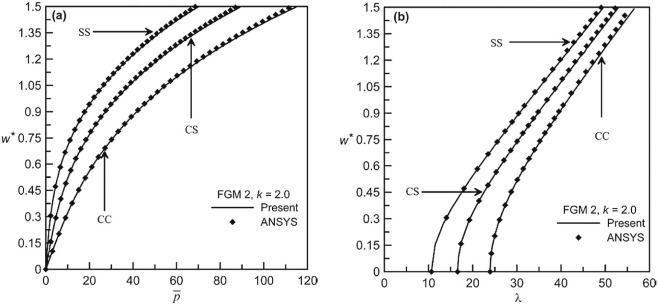Fig. 2. Validation plots of pre-stressed beam: (a) static behavior and (b) free vibration behavior.

### 3.2. Natural frequency of vibration of undeformed FGM beam

The non-dimensional frequency parameter ${\textstyle \lambda }$ of undeformed CC beam for all the four functionally graded materials considered is presented in Table 5 for different volume fraction indices. The list presented in Table 5 includes results for L/h  = 10 and L/h  = 25. Similar lists for SS and CS FGM beams are presented in Table 6  and Table 7 respectively. As seen from these tables, the non-dimensional frequency parameter ${\textstyle \lambda }$ decreases with increase in values of k , and this is true for all the four FGMs considered. Only exception to this occurs for FGM 2 with L/h    = 25, where ${\textstyle \lambda }$ increases from k  = 20 to k  = 50. It is to be mentioned that higher k values indicate more metal content in the beam. It is also seen that the non-dimensional frequency parameter increases with L/h ratio but the change is very little. The effect of L/h ratio on non-dimensional frequency of vibration remains insignificant for pre-stressed beam also. Hence the results, as presented in the following section, for pre-stressed FGM beams are generated for L/h  = 25.

Table 5. List of non-dimensional frequency parameters ${\textstyle \lambda }$ for CC beam.
k L/h  = 10 L/h  = 25
FGM 1 FGM 2 FGM 3 FGM 4 FGM 1 FGM 2 FGM 3 FGM 4
0.0 48.595 31.128 38.477 32.142 51.212 32.872 40.537 33.943
0.1 43.041 29.308 35.828 30.774 45.334 30.915 37.723 32.470
0.2 39.411 28.051 33.864 29.715 41.448 29.597 35.751 31.378
0.5 33.475 25.815 30.290 27.617 35.312 27.260 31.870 29.106
1.0 29.326 24.070 27.502 25.862 30.803 25.413 28.969 27.268
2.0 26.288 22.657 25.320 24.425 27.742 23.896 26.728 25.802
5.0 23.853 21.563 23.445 23.102 25.246 22.712 24.771 24.430
10.0 22.697 21.198 22.499 22.358 24.001 22.348 23.799 23.581
20.0 21.953 21.052 21.847 21.802 23.204 22.190 23.099 23.055
50.0 21.390 20.984 21.350 21.350 22.579 22.135 22.552 22.547

Table 6. List of non-dimensional frequency parameters ${\textstyle \lambda }$ for SS beam.
k L/h  = 10 L/h  = 25
FGM 1 FGM 2 FGM 3 FGM 4 FGM 1 FGM 2 FGM 3 FGM 4
0.0 22.457 14.410 17.789 14.880 22.393 14.596 17.804 15.072
0.1 19.873 13.573 16.532 14.234 20.200 13.920 16.718 14.514
0.2 18.208 12.998 15.637 13.759 18.303 13.270 15.796 14.043
0.5 15.522 11.969 14.046 12.829 15.692 12.186 14.249 12.915
1.0 13.635 11.159 12.794 12.048 13.772 11.279 12.989 12.230
2.0 12.243 10.500 11.803 11.399 12.250 10.472 12.031 11.613
5.0 11.098 9.999 10.909 10.746 11.234 10.140 11.133 10.924
10.0 10.566 9.819 10.461 10.401 10.561 9.986 10.586 10.497
20.0 10.168 9.747 10.147 10.119 10.258 9.811 10.310 10.286
50.0 9.934 9.704 9.886 9.889 10.042 9.873 10.152 9.953

Table 7. List of non-dimensional frequency parameters ${\textstyle \lambda }$ for CS beam.
k L/h  = 10 L/h  = 25
FGM 1 FGM 2 FGM 3 FGM 4 FGM 1 FGM 2 FGM 3 FGM 4
0.0 34.326 22.013 27.203 22.730 35.330 22.785 28.021 23.527
0.1 30.411 20.740 25.306 21.783 31.326 21.558 25.969 22.512
0.2 27.831 19.853 23.940 21.017 29.007 20.403 24.488 21.737
0.5 23.681 18.276 21.416 19.527 24.481 18.867 22.181 19.990
1.0 20.728 17.036 19.472 18.310 21.457 17.507 20.152 18.861
2.0 18.622 16.034 17.934 17.298 19.166 16.534 18.662 18.033
5.0 16.889 15.252 16.615 19.373 17.299 15.773 17.022 17.098
10.0 16.094 14.998 15.934 15.845 16.395 15.463 16.504 16.321
20.0 15.553 14.899 15.482 15.444 19.120 15.330 16.050 16.026
50.0 15.146 14.842 15.113 15.112 15.649 15.321 15.572 15.662

### 3.3. Effect of material and volume fraction index on static and dynamic behavior

The static deflection behavior of CC beam in ${\textstyle {\overline {p}}-w\ast }$ plane is presented in Fig. 3 (a–d) for FGM 1, FGM 2, FGM 3 and FGM 4 respectively. In each of these figures, the static equilibrium path is presented for a set of volume fraction indices, i.e., for k  = 0.0, 0.1, 0.2, 0.5, 1.0, 2.0, 5.0, 10.0, 20.0, 50.0. The plots are useful in finding the non-dimensional pressure value corresponding to a static deflection level, at which the loaded natural frequency is found out. As seen from Fig. 3 , the beam exhibits non-linear hardening type load-deflection behavior. This is due to stiffening effect induced in the beam as a result of generation of tensile membrane forces due to immovable ends. With increase in k , i.e., the increase in metal content, FGM 1, FGM 3 and FGM 4 beam shows decreased stiffness levels as the elastic modulus of ceramic constituent is greater than its metal counterpart, as can be seen from Table 3 . The trend is completely reverse for FGM 2 beam as the elastic modulus of its ceramic constituent is lesser than that of its metal part.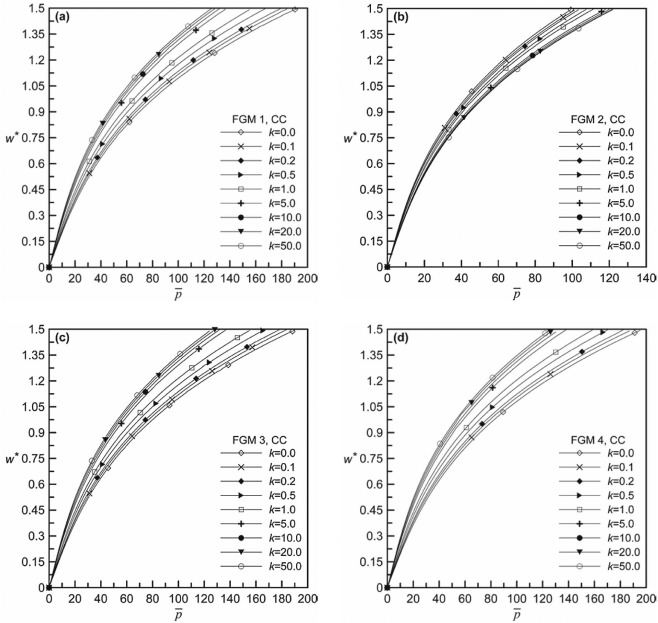Fig. 3. Non-dimensional pressure-deflection behavior for different volume fraction indices of CC beams: (a) FGM 1, (b) FGM 2, (c) FGM 3 and (d) FGM 4.

The loaded natural frequency versus maximum transverse deflection plots in non-dimensional ${\textstyle \lambda -w\ast }$ plane is presented in Fig. 4 (a–f) for k  = 0.0, 0.2, 0.5, 5.0, 20.0 and 50.0, respectively, for CC FGM beam. In each of the figures, free vibration behavior is shown for FGM 1, FGM 2, FGM 3 and FGM 4 beams. In accordance with the static behavior, the loaded natural frequency is shown to be increasing with increased deflection level as a result of enhanced stiffening effect. With regard to the comparative behavior among different FGMs considered, FGM 1 shows highest frequency of vibration with FGM 3, FGM 4 and FGM 2 coming next in order of exhibiting decreasing vibration frequency at any common deflection levels. This is true irrespective of the values of the volume fraction index. It is also seen that the relative differences in frequency-deflection behavior of various FGMs diminish with increase in k values. At higher k values, the dynamic behavior becomes almost identical for all the FGMs considered.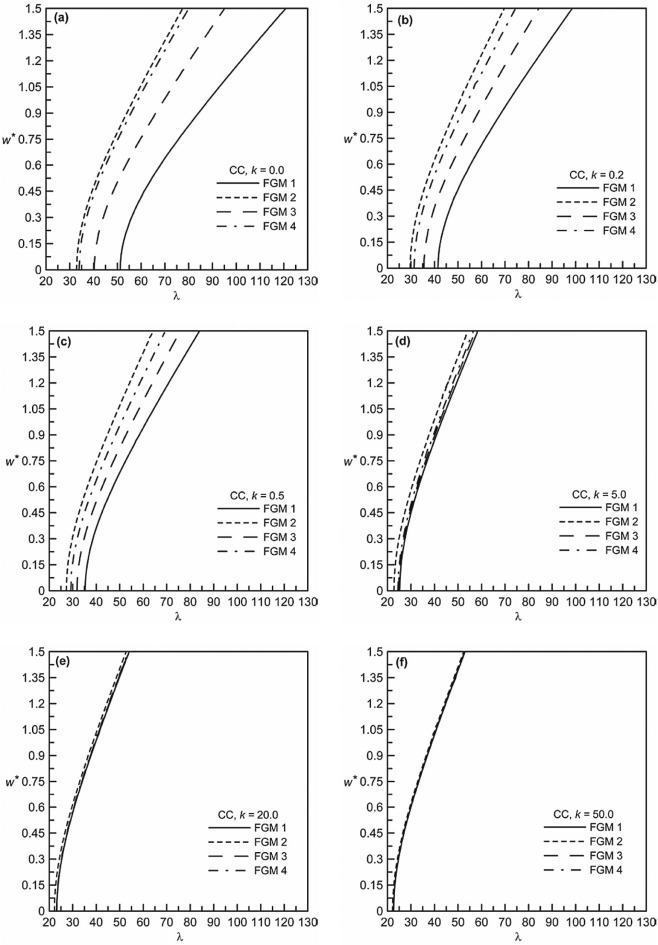Fig. 4. Non-dimensional frequency-deflection behavior of different CC FGM beams: (a) k  = 0.0, (b) k  = 0.2, (c) k  = 0.5, (d) k  = 5.0, (e) k  = 20.0 and (f) k  = 50.0.

It is also important to study the dynamic behavior of pre-stressed FGM beam for different volume fraction indices. Fig. 5 (a–d) shows such non-dimensional frequency-deflection plots for FGM 1. FGM 2, FGM 3 and FGM 4, respectively, each showed comparative behavior for k  = 0.0, 0.1, 1.0, 10.0, 50.0. It can be seen that the loaded natural frequency decreases with increase in k values for any particular deflection level. This is true for all the FGMs considered. The explanation for the variation of loaded natural frequency with k cannot be given by seeing only the static deflection behavior as shown in Fig. 3 . Because in predicting the dynamic behavior, both the relative density values and the relative elastic modulus values of the constituents are to be considered.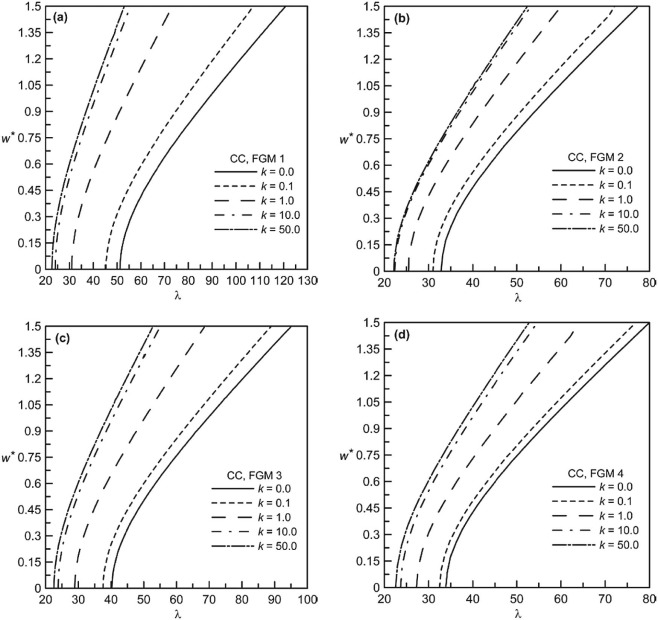Fig. 5. Non-dimensional frequency-deflection behavior of CC beams for different volume fraction indices: (a) FGM 1, (b) FGM 2, (c) FGM 3 and (d) FGM 4.

The non-dimensional static deflection behavior for different volume fraction indices is presented in Fig. 6 (a–d) for SS beams each for different functionally graded materials. Also the non-dimensional frequency-deflection plots of SS beams are shown in Fig. 7 (a–f) for different k values and in Fig. 8 (a–d) for different FGMs. As for CS beams, static deflection behavior is presented in Fig. 9 (a–d), whereas the dynamic behavior in terms of frequency-deflection plots is shown in Fig. 10 (a–f) for different k values and in Fig. 11 (a–d) for different FGMs. For both these boundary conditions, the nature of static and dynamic behavior is similar in nature as described for CC beams. The relative behavior for these three different boundary conditions, although not shown in a single plot, differs obviously due to the stiffness effects contributed from the support conditions. Because it is known that CC beam exhibits the highest transverse stiffness, with CS and SS beam being next in order of decreasing stiffness levels.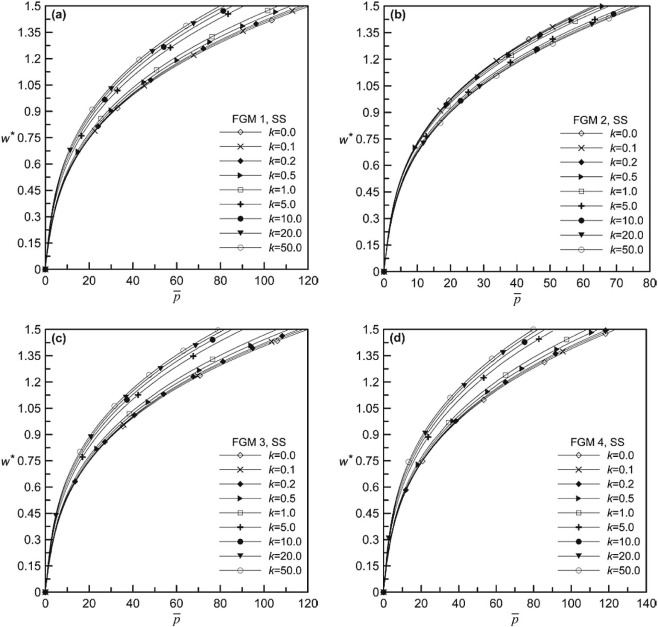Fig. 6. Non-dimensional pressure-deflection behavior for different volume fraction indices of SS beam: (a) FGM 1, (b) FGM 2, (c) FGM 3 and (d) FGM 4.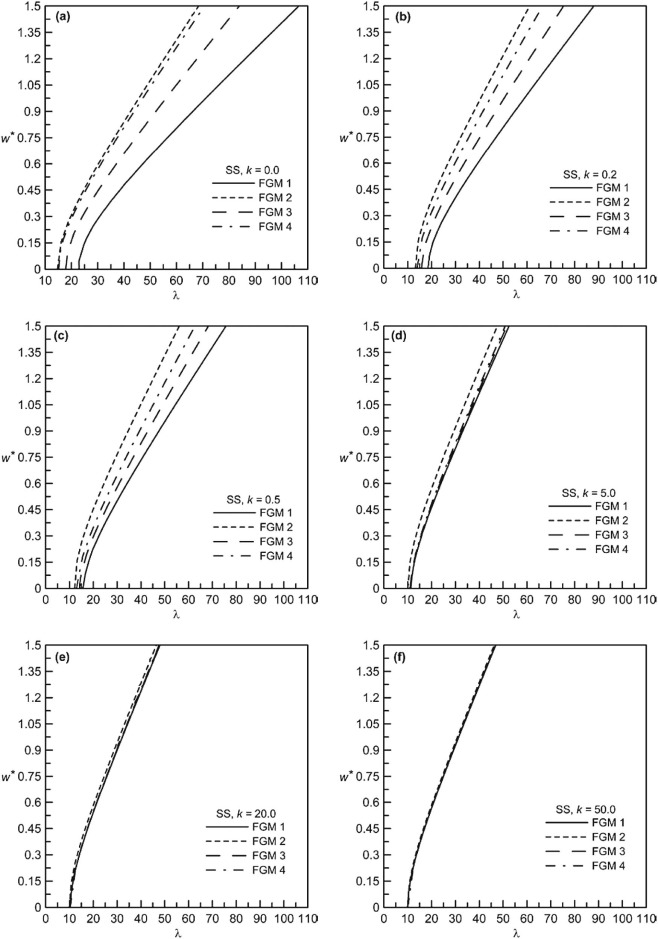Fig. 7. Non-dimensional frequency-deflection behavior of different SS FGM beams: (a) k  = 0.0, (b) k  = 0.2, (c) k  = 0.5, (d) k  = 5.0, (e) k  = 20.0 and (f) k  = 50.0.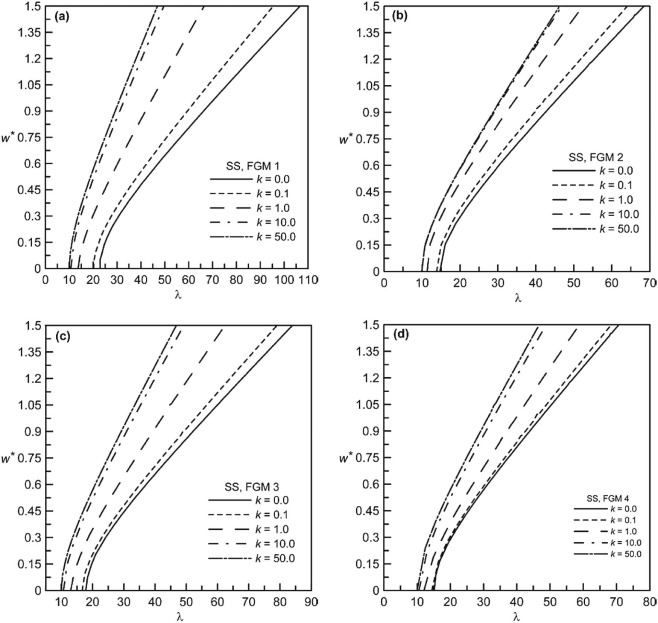Fig. 8. Non-dimensional frequency-deflection behavior of SS beams for different volume fraction indices: (a) FGM 1, (b) FGM 2, (c) FGM 3 and (d) FGM 4.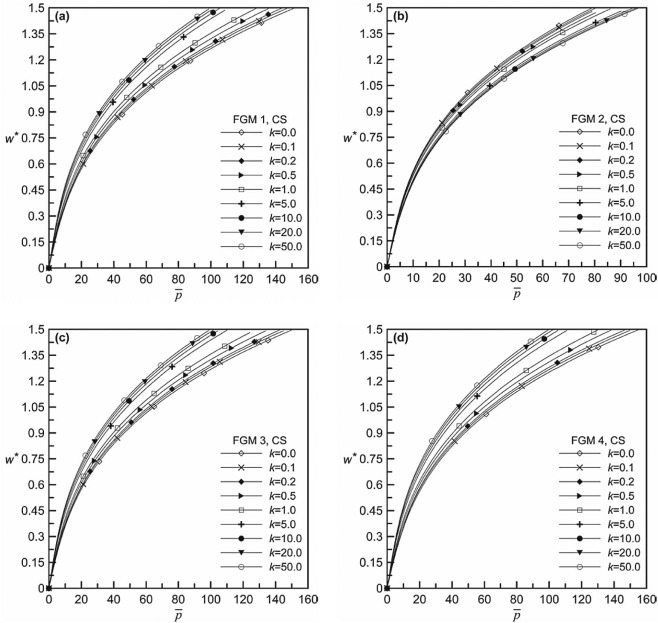Fig. 9. Non-dimensional pressure-deflection behavior for different volume fraction indices of CS beam: (a) FGM 1, (b) FGM 2, (c) FGM 3 and (d) FGM 4.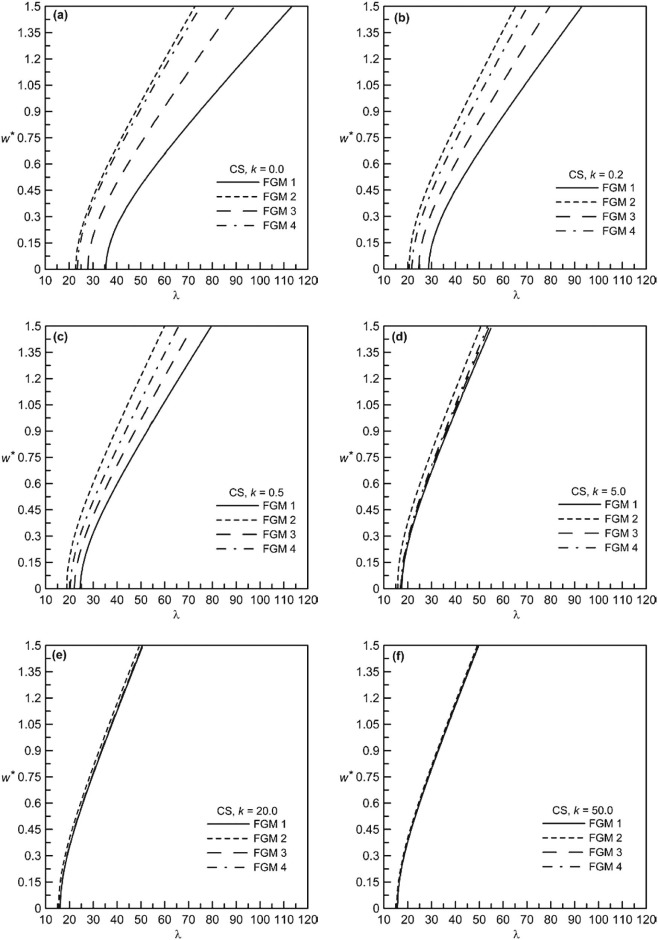Fig. 10. Non-dimensional frequency-deflection behavior of different CS FGM beams: (a) k  = 0.0, (b) k  = 0.2, (c) k  = 0.5, (d) k  = 5.0, (e) k  = 20.0 and (f) k  = 50.0.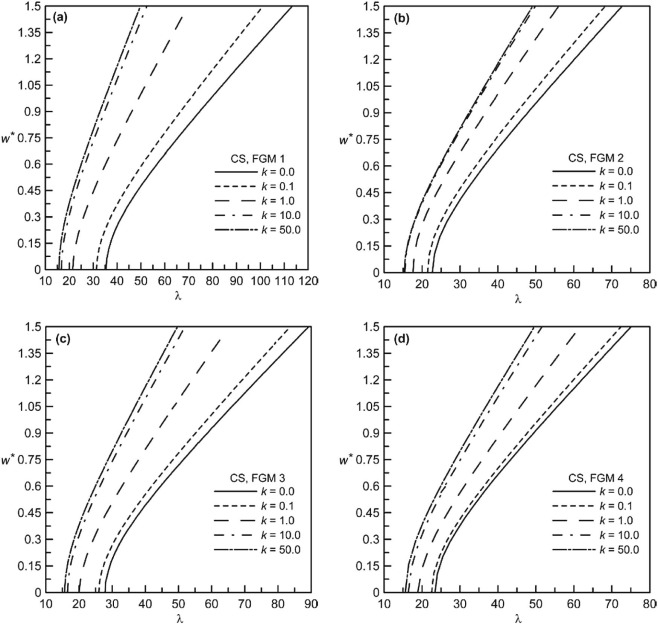Fig. 11. Non-dimensional frequency-deflection behavior of CS beams for different volume fraction indices: (a) FGM 1, (b) FGM 2, (c) FGM 3 and (d) FGM 4.

## 4. Conclusions

An energy based mathematical model is presented to study the free vibration behavior of pre-stressed FGM Timoshenko beams. The entire work is carried out in solving two different but interrelated problems, namely the static problem and the dynamic problem. The static problem is used to determine the pre-stressed configuration of FGM beam under uniform transverse pressure. And the dynamic problem, formulated as an eigenvalue problem, is used to determine the loaded natural frequency of the pre-stressed beam. Four different FGMs, namely Stainless Steel–Silicon Nitride, Stainless Steel–Zirconia, Stainless Steel–Alumina and Titanium alloy–Zirconia, are used to generate results for different volume fraction indices. Numerical results for non-dimensional frequency parameters of undeformed beam are presented for different functionally graded materials with CC, SS and CS boundary conditions. Effects of material as well as the volume fraction index on non-dimensional frequency-deflection behavior of pre-stressed beams are studied. The results are presented for three boundary conditions, i.e., CC, SS and CS. Static equilibrium paths in non-dimensional plane are also presented in order to relate the applied pressure with loaded natural frequency through the static deflection level. The results can serve as benchmarks for further study in this field.

## Elements of stiffness matrix and load vector

 ${\left[k_{\mbox{ij}}\right]}_{\begin{array}{c}i=1,nw\\j=1,nw\end{array}}={\frac {A_{ax}}{2}}\int _{0}^{L}{\left(\sum _{k=1}^{nw}d_{k}{\frac {d{\phi }_{k}}{dx}}\right)}^{2}{\frac {d{\phi }_{i}}{dx}}{\frac {d{\phi }_{j}}{dx}}dx+$$A_{ax}\int _{0}^{L}\left(\sum _{k=nw+1}^{nw+nu}d_{k}{\frac {d{\alpha }_{k-nw}}{dx}}\right){\frac {d{\phi }_{i}}{dx}}{\frac {d{\phi }_{j}}{dx}}dx-$$B_{ax}\int _{0}^{L}\left(\sum _{k=nw+nu+1}^{nw+nu+nsi}d_{k}{\frac {d{\beta }_{k-nw-nu}}{dx}}\right){\frac {d{\phi }_{i}}{dx}}{\frac {d{\phi }_{j}}{dx}}dx$ ${\mbox{ }}+{\mbox{ }}k_{sh}A_{sh}\int _{0}^{L}{\frac {d{\phi }_{i}}{dx}}{\frac {d{\phi }_{j}}{dx}}dx,{\mbox{ }}\cdots {\mbox{ }}{\left[k_{\mbox{ij}}\right]}_{\begin{array}{c}i=1,nw\\j=nw+1,nw+nu\end{array}}=0,$
 ${\left[k_{\mbox{ij}}\right]}_{\begin{array}{c}i=1,nw\\j=nw+nu+1,nw+nu+nsi\end{array}}=-k_{sh}A_{sh}\int _{0}^{L}{\frac {d{\phi }_{i}}{dx}}{\beta }_{j-nw-nu}dx,{\mbox{ }}{\left[k_{\mbox{ij}}\right]}_{\begin{array}{c}i=nw+1,nw+nu\\j=1,nw\end{array}}={\frac {A_{ax}}{2}}\int _{0}^{L}\left(\sum _{k=1}^{nw}d_{k}{\frac {d{\phi }_{k}}{dx}}\right){\frac {d{\alpha }_{i-nw}}{dx}}{\frac {d{\phi }_{j}}{dx}}dx,$
 ${\left[k_{\mbox{ij}}\right]}_{\begin{array}{c}i=nw+1,nw+nu\\j=nw+1,nw+nu\end{array}}=A_{ax}\int _{0}^{L}{\frac {d{\alpha }_{i-nw}}{dx}}{\frac {d{\alpha }_{j-nw}}{dx}}dx,{\mbox{ }}{\left[k_{\mbox{ij}}\right]}_{\begin{array}{c}i=nw+1,nw+nu\\j=nw+nu+1,nw+nu+nsi\end{array}}=-B_{ax}\int _{0}^{L}{\frac {d{\alpha }_{i-nw}}{dx}}{\frac {d{\beta }_{j-nw-nu}}{dx}}dx,$
 ${\left[k_{\mbox{ij}}\right]}_{\begin{array}{c}i=nw+nu+1,nw+nu+nsi\\j=1,nw\end{array}}=-{\frac {B_{ax}}{2}}\int _{0}^{L}\left(\sum _{k=1}^{nw}d_{k}{\frac {d{\phi }_{k}}{dx}}\right){\frac {d{\beta }_{i-nw-nu}}{dx}}{\frac {d{\phi }_{j}}{dx}}dx-$$k_{sh}A_{sh}\int _{0}^{L}{\beta }_{i-nw-nu}{\frac {d{\phi }_{j}}{dx}}dx,$
 ${\left[k_{\mbox{ij}}\right]}_{\begin{array}{c}i=nw+nu+1,nw+nu+nsi\\j=nw+1,nw+nu\end{array}}=-B_{ax}\int _{0}^{L}{\frac {d{\beta }_{i-nw-nu}}{dx}}{\frac {d{\alpha }_{j-nw}}{dx}}dx,$
 ${\left[k_{\mbox{ij}}\right]}_{\begin{array}{c}i=nw+nu+1,nw+nu+nsi\\j=nw+nu+1,nw+nu+nsi\end{array}}=C_{ax}\int _{0}^{L}{\frac {d{\beta }_{j-nw-nu}}{dx}}{\frac {d{\beta }_{i-nw-nu}}{dx}}dx+$$k_{sh}A_{sh}\int _{0}^{L}{\beta }_{j-nw-nu}{\beta }_{i-nw-nu}dx.$
 ${\left\{f_{i}\right\}}_{i=1,nw}=p\int _{0}^{L}{\phi }_{i}dx,{\mbox{ }}{\left\{f_{i}\right\}}_{i=nw+1,nw+nu}=$$0,{\mbox{ }}{\left\{f_{i}\right\}}_{i=nw+nu+1,nw+nu+nsi}=$$0.$

### Document informationPublished on 10/04/17

Licence: Other

### Document Score4

Views 79
Recommendations 1